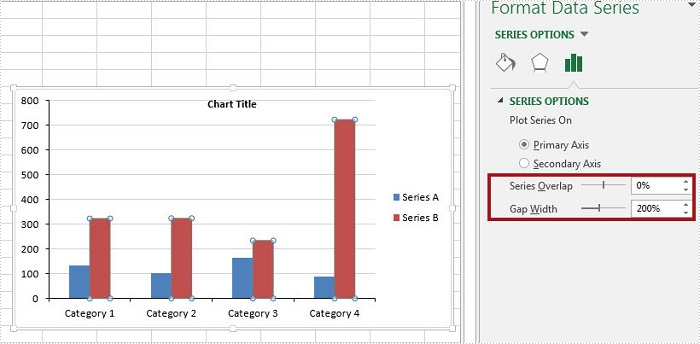# How to Adjust the Spaces between Bars in Excel Chart in C#, VB.NET

In MS Excel, the spaces between data bars have been defined as Series Overlap and Gap Width.

• Series Overlap: Spaces between data series within a single category.
• Gap Width: Spaces between two categories.

Check below picture, you'll have a better understanding of these two concepts. Normally the spaces are automatically calculated based on the date and chart area, the space may be very narrow or wide depending on how many date series you have in a fixed chart area. In this article, we'll introduce how to adjust the spaces between data bars using Spire.XLS.Code Snippet:

Step 1: Initialize a new instance of Wordbook class and load the sample Excel file that contains some data in A1 to C5.

```Workbook workbook = new Workbook();
```

Step 2: Create a Column chart based on the data in cell range A1 to C5.

```Worksheet sheet = workbook.Worksheets;
chart.DataRange = sheet.Range["A1:C5"];
chart.SeriesDataFromRange = false;
chart.PrimaryValueAxis.MajorGridLines.LineProperties.Color = Color.LightGray;
```

Step 3: Set chart position.

```chart.LeftColumn = 5;
chart.TopRow = 7;
chart.RightColumn = 13;
chart.BottomRow = 21;
```

Step 4: The ChartSerieDataFormat class has two properties - GapWidth property and Overlap property to handle the Gap Width and Series Overlap respectively. The value of GapWidth varies from 0 to 500, and the value of Overlap varies from -100 to 100.

```foreach (ChartSerie cs in chart.Series)
{
cs.Format.Options.GapWidth = 200;
cs.Format.Options.Overlap = 0;
}
```

Step 5: Save and launch the file.

```workbook.SaveToFile("result.xlsx", ExcelVersion.Version2010);
System.Diagnostics.Process.Start("result.xlsx");
```

Output:Full Code:

[C#]
```using Spire.Xls;
using Spire.Xls.Charts;
{

class Program
{

static void Main(string[] args)
{
Workbook workbook = new Workbook();

Worksheet sheet = workbook.Worksheets;
chart.DataRange = sheet.Range["A1:C5"];
chart.SeriesDataFromRange = false;
chart.PrimaryValueAxis.MajorGridLines.LineProperties.Color = Color.LightGray;

chart.LeftColumn = 5;
chart.TopRow = 7;
chart.RightColumn = 13;
chart.BottomRow = 21;

foreach (ChartSerie cs in chart.Series)
{
cs.Format.Options.GapWidth = 200;
cs.Format.Options.Overlap = 0;
}

workbook.SaveToFile("result.xlsx", ExcelVersion.Version2010);
System.Diagnostics.Process.Start("result.xlsx");

}
}
}
```
[VB.NET]
```Imports Spire.Xls
Imports Spire.Xls.Charts

Class Program

Private Shared Sub Main(args As String())
Dim workbook As New Workbook()

Dim sheet As Worksheet = workbook.Worksheets(0)
Dim chart As Chart = sheet.Charts.Add(ExcelChartType.ColumnClustered)
chart.DataRange = sheet.Range("A1:C5")
chart.SeriesDataFromRange = False
chart.PrimaryValueAxis.MajorGridLines.LineProperties.Color = Color.LightGray

chart.LeftColumn = 5
chart.TopRow = 7
chart.RightColumn = 13
chart.BottomRow = 21

For Each cs As ChartSerie In chart.Series
cs.Format.Options.GapWidth = 200
cs.Format.Options.Overlap = 0
Next

workbook.SaveToFile("result.xlsx", ExcelVersion.Version2010)
System.Diagnostics.Process.Start("result.xlsx")

End Sub
End Class
End Namespace
```